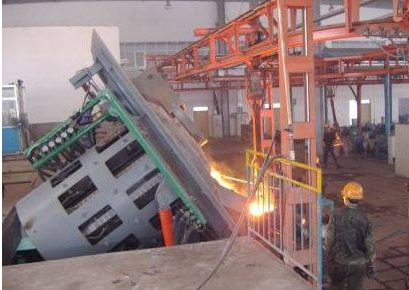### Calculation method of thyristor selection for induction melting furnace

Calculation method of thyristor selection for induction melting furnace

Selection of thyristor current value calculated by induction melting furnace:

A. Calculate the rectified DC voltage according to the incoming line voltage

DC voltage Vd=1.35×incoming line voltage

DC voltage Vd=1.26×incoming line voltage

B. DC current

DC current Id max = intermediate frequency power / DC voltage Vd

DC current Id max=0.5×intermediate frequency power/DC voltage Vd

c. The average current of the rectifier SCR can be calculated as follows

Ikpmax=Id max／2.72

d. The average current of the inverter thyristor can be calculated by the following formula

Ikkmax=0.45×Idmax

Through the above formula, select the specifications of the thyristor and select them to have a safety factor greater than 2 times.

The selection of the voltage value of the thyristor calculated by the induction melting furnace:

A. The tolerable peak voltage Vkp on the rectifier SCR = 1.414× the incoming line voltage Vi

B. The tolerable peak voltage on the inverter thyristor Vkk=1.414×the intermediate frequency voltage Vo

Among them, the intermediate frequency voltage Vo=1.5×DC voltage Vd (Vd has been calculated before)

According to the calculated peak voltage Vkp and Vkk tolerated on the rectifier and inverter thyristor, when selecting the thyristor, the withstand voltage value of the thyristor should be greater than twice the withstand peak voltage of the thyristor Select.